### Go Map 原理

map 的底层实现是一个散列表，因此实现 map 的过程实际上就是实现散表的过程。

hmap: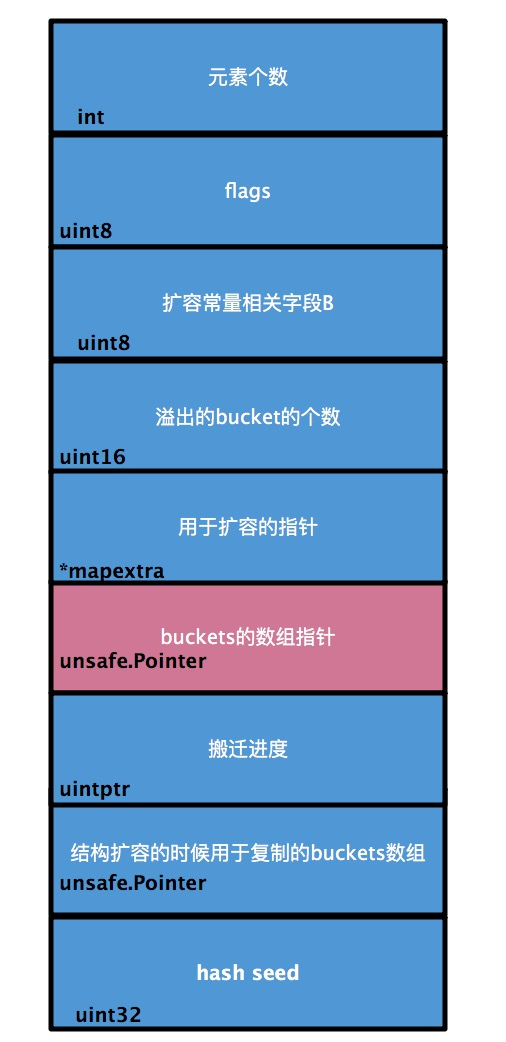bucket：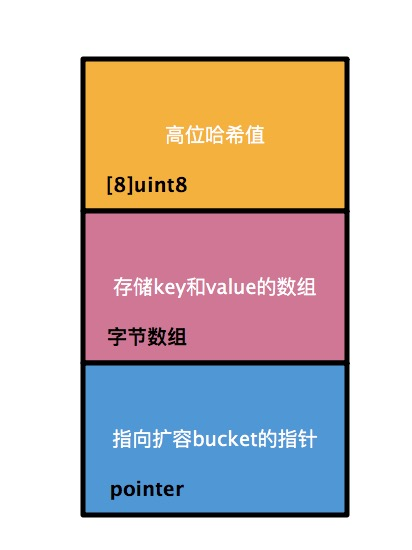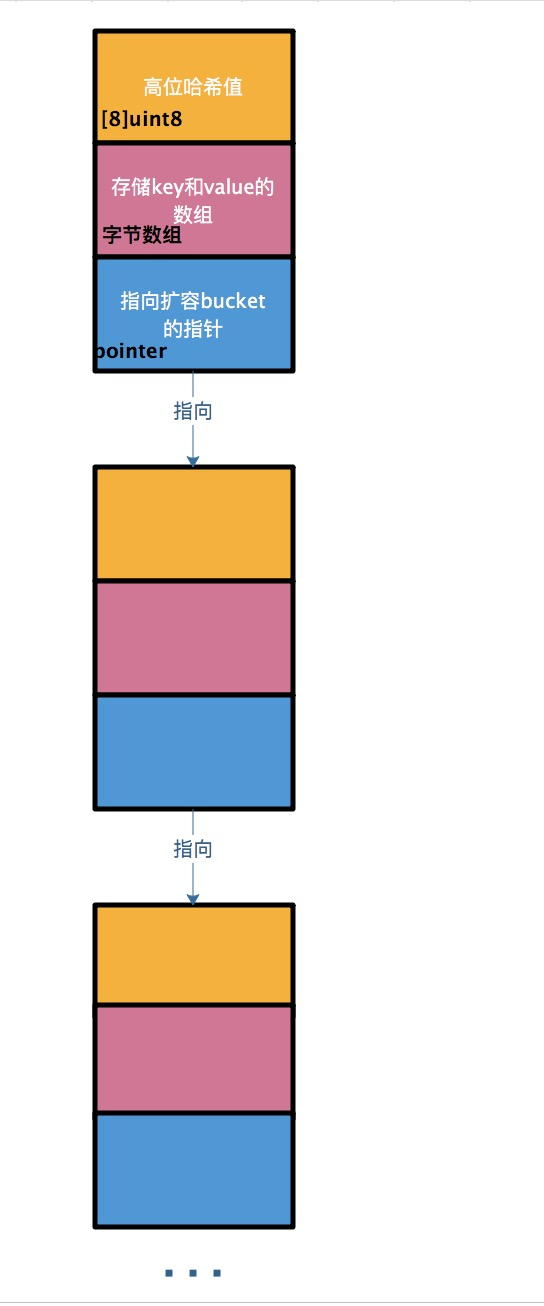Go 把求得的值按照用途一分为二：高位和低位。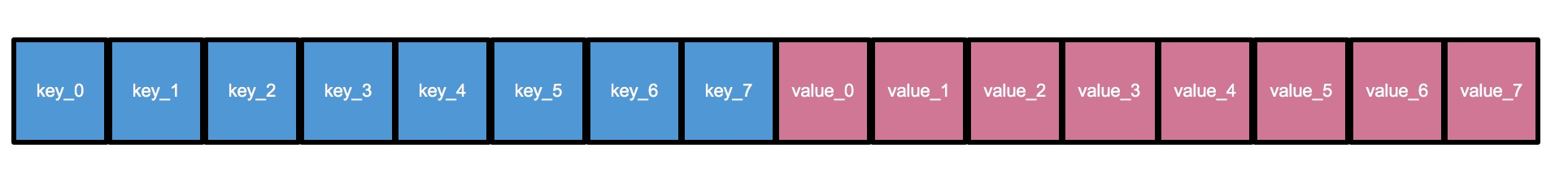map整个的结构图### 加载因子

``map长度 / 2^B``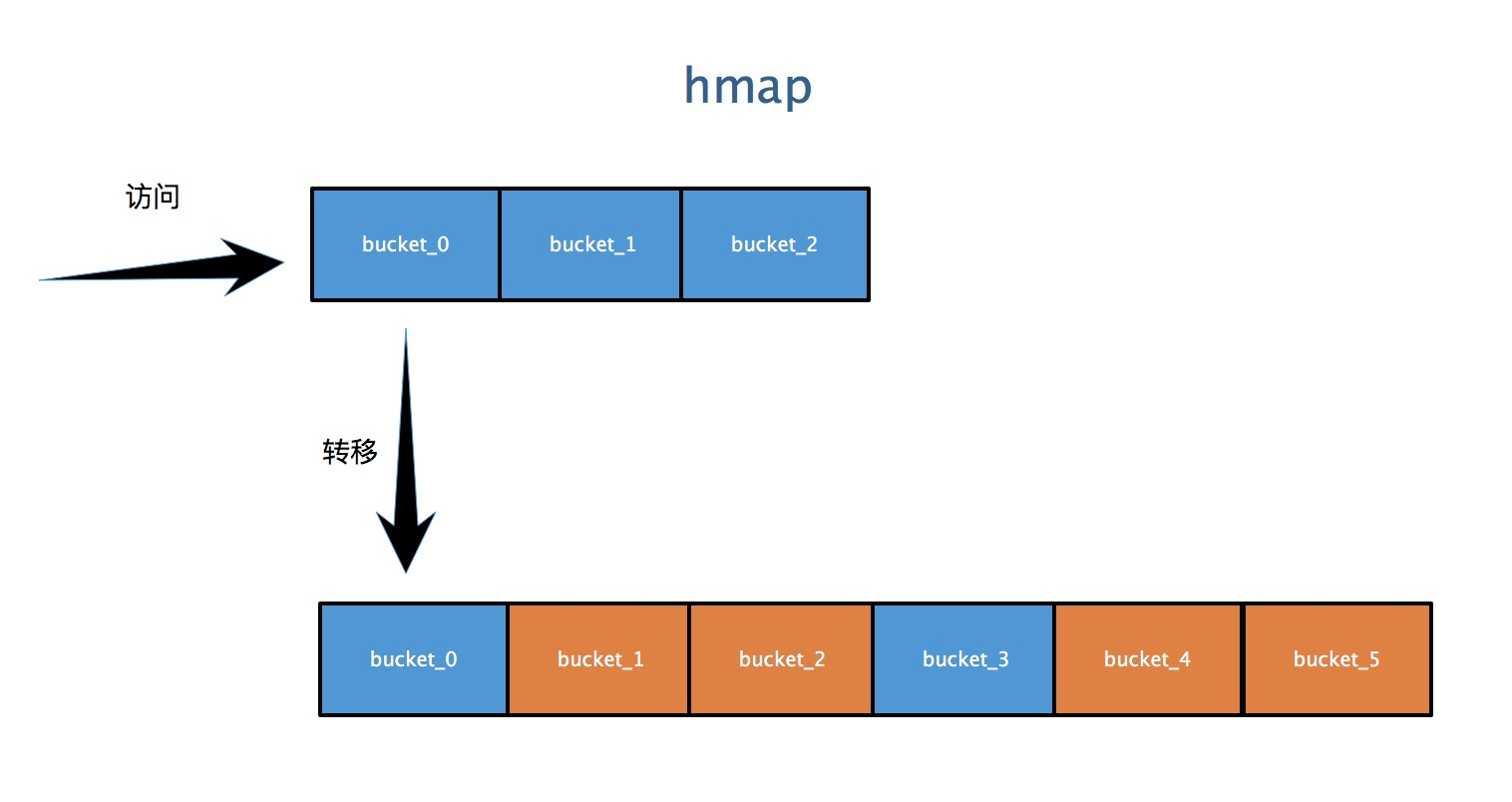### map 中数据的删除

1. 如果 `key` 是一个指针类型的，则直接将其置为空，等待 GC 清除。
2. 如果是值类型的，则清除相关内存。
3. 同理，对 `value` 做相同的操作。
4. 最后把 `key` 对应的高位值对应的数组 index 置为空。

### Go Map

``var m1 map[string]string``

make 函数会分配和初始化一个哈希 map 数据结构，并返回一个指向该哈希 map 的映射变量。相关数据结构细节由运行时实现，并且不因语言本身所规定。

``m1 = make(map[string]string)``

``````m1["a"] = "aa"
m1["b"] = "bb"``````

### Go 比较两个 Slice 是否相等

• reflect 比较的方法
• 循环遍历比较的方法

reflect 比较的方法

``````func StringSliceReflectEqual(a, b []string) bool {
return reflect.DeepEqual(a, b)
}``````

### Array

Go 语言的数组不同于 C 语言或者其他语言的数组，C 语言的数组变量是指向数组第一个元素的指针；而 Go 语言的数组是一个值，Go 语言中的数组是值类型，一个数组变量就表示着整个数组，意味着 Go 语言的数组在传递的时候，传递的是原数组的拷贝。你可以理解为 Go 语言的数组是一种有序的 struct。

``````func testArray(x int) {
fmt.Printf("func Array : %p , %v\n", &x, x)
}

func main() {
arrayA := int{100, 200}
var arrayB int
arrayB = arrayA
fmt.Printf("arrayA : %p , %v\n", &arrayA, arrayA)
fmt.Printf("arrayB : %p , %v\n", &arrayB, arrayB)
testArray(arrayA)
}``````

``````arrayA : 0xc4200bebf0 , [100 200]
arrayB : 0xc4200bec00 , [100 200]
func Array : 0xc4200bec30 , [100 200]``````

### 数组### slice

``````package main

import (
"fmt"
)

func main() {
var x = make([]float64, 5)
fmt.Println("Capcity:", cap(x), "Length:", len(x))
var y = make([]float64, 5, 10)
fmt.Println("Capcity:", cap(y), "Length:", len(y))
for i := 0; i < len(x); i++ {
x[i] = float64(i)
}
fmt.Println(x)
for i := 0; i < len(y); i++ {
y[i] = float64(i)
}
fmt.Println(y)
}``````

``````Capcity: 5 Length: 5
Capcity: 10 Length: 5
[0 1 2 3 4]
[0 1 2 3 4]``````

``````package main

import (
"fmt"
)

func main() {
var arr1 = int{1, 2, 3, 4, 5}
var s1 = arr1[2:3]
var s2 = arr1[:3]
var s3 = arr1[2:]
var s4 = arr1[:]
fmt.Println(s1)
fmt.Println(s2)
fmt.Println(s3)
fmt.Println(s4)
}``````

``````
[1 2 3]
[3 4 5]
[1 2 3 4 5]``````

``````package main

import (
"fmt"
)

func main() {
var arr1 = make([]int, 5, 10)
for i := 0; i < len(arr1); i++ {
arr1[i] = i
}
fmt.Println(arr1)
arr1 = append(arr1, 5, 6, 7, 8)
fmt.Println("Capacity:", cap(arr1), "Length:", len(arr1))
fmt.Println(arr1)
}``````

``````[0 1 2 3 4]
Capacity: 10 Length: 9
[0 1 2 3 4 5 6 7 8]``````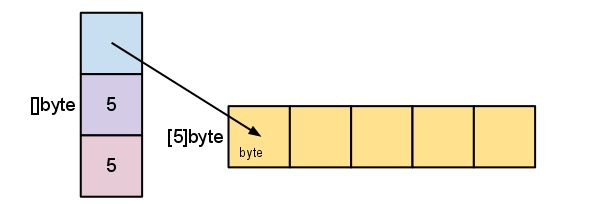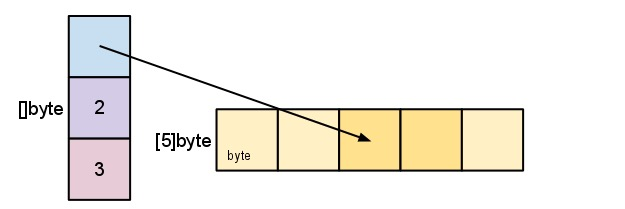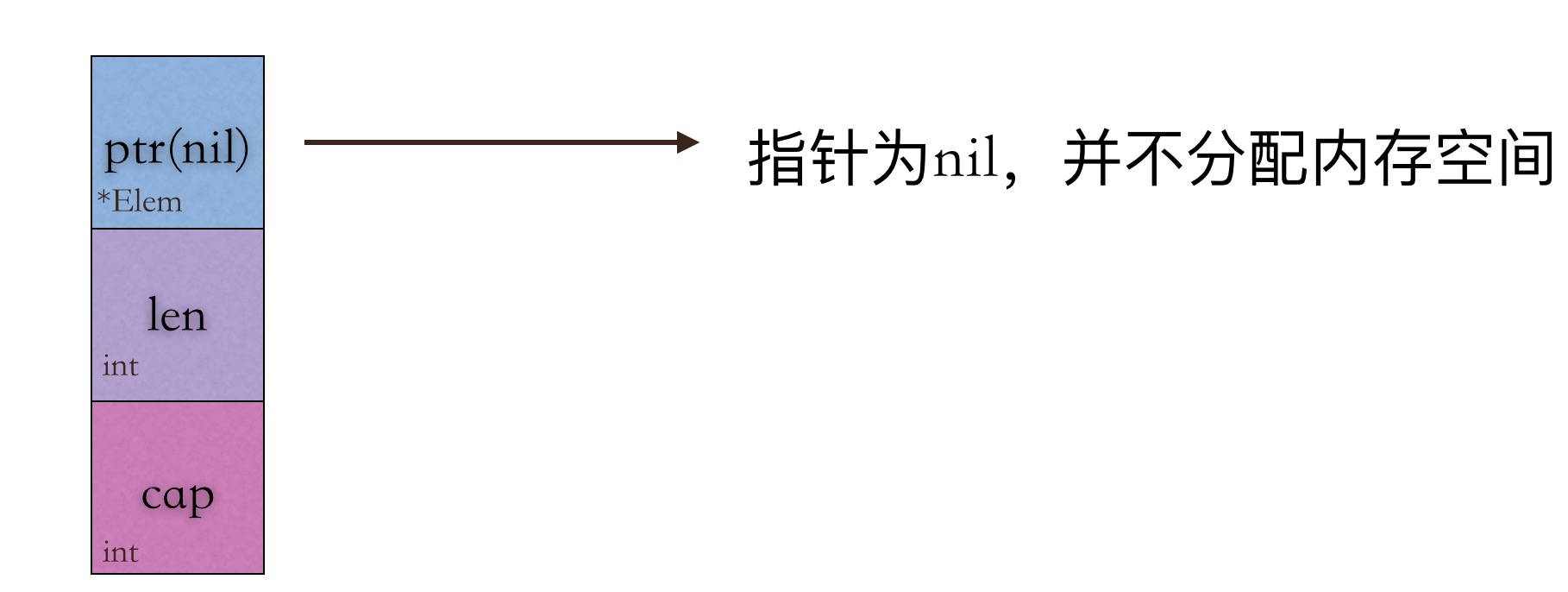### 扩容

``````package main

import (
"fmt"
)

func main() {
var arr1 = make([]int, 5, 10)
for i := 0; i < len(arr1); i++ {
arr1[i] = i
}
arr1 = append(arr1, 5, 6, 7, 8, 9, 10)
fmt.Println("Capacity:", cap(arr1), "Length:", len(arr1))
fmt.Println(arr1)
}``````

``````Capacity: 20 Length: 11
[0 1 2 3 4 5 6 7 8 9 10]``````

``````package main

import (
"fmt"
)

func main() {
slice1 := []int{1, 2, 3, 4, 5, 6}
slice2 := make([]int, 5, 10)
copy(slice2, slice1)
fmt.Println(slice1)
fmt.Println(slice2)
}``````

``````[1 2 3 4 5 6]
[1 2 3 4 5]``````

### 应用

``````package main

import (
"fmt"
"reflect"
)

func main() {
// index
arrayA := [...]int{0: 1, 2: 1, 3: 4}
fmt.Println(arrayA)

// type
arrayB := [...]int{1, 2, 3}
arrayC := [...]int{1, 2, 3, 4}
fmt.Println(reflect.TypeOf(arrayB))
fmt.Println(reflect.TypeOf(arrayC))
fmt.Println(reflect.TypeOf(arrayB) == reflect.TypeOf(arrayC))

array := int{10, 20, 30, 40}
slice := array[0:2]
fmt.Println(slice)

newSlice := append(append(append(slice, 50), 100), 150)
fmt.Println(newSlice)

newSlice = newSlice + 1
fmt.Println(newSlice)

sliceInt := []int{1, 2, 3, 4, 5, 6}
newSliceInt := append(sliceInt, 1)
fmt.Println(newSliceInt)
}``````
``r := [...]int{99:-1}``

### Go base64

``````package main

import (
"encoding/base64"
"fmt"
"log"
)

func main() {
input := []byte("hello golang base64")

// base64 encode
encodeString := base64.StdEncoding.EncodeToString(input)
fmt.Println(encodeString)

// base decode
decodeBytes, err := base64.StdEncoding.DecodeString(encodeString)
if err != nil {
log.Fatalln(err)
}
fmt.Println(string(decodeBytes))
}``````

### Go 计算字符串 md5 值

``````package utils

import (
"crypto/md5"
"encoding/hex"
)

// MakeMD func
func MakeMD(initString string) string {
m := md5.New()
m.Write([]byte(initString))
md := m.Sum(nil)
mdString := hex.EncodeToString(md)
return mdString
}``````

### Go 字符串分割

``````str := "Hello World Too"
for _, v := range strings.Fields(str) {
fmt.Println(v)
}``````

``````for _, v := range strings.Split(str, " ") {
fmt.Println(v)
}``````

``````for _, v := range strings.SplitAfter(str, ",") {
fmt.Println(v)
}``````

### Go 字符串拼接

1.直接使用运算符

``````func BenchmarkAddStringWithOperator(b *testing.B) {
hello := "hello"
world := "world"
for i := 0; i < b.N; i++ {
_ = hello + "," + world
}
}``````

go 里面的字符串都是不可变的，每次运算都会产生一个新的字符串，所以会产生很多临时的无用的字符串，不仅没有用，还会给 gc 带来额外的负担，所以性能比较差。

2.fmt.Sprintf()

``````func BenchmarkAddStringWithSprintf(b *testing.B) {
hello := "hello"
world := "world"
for i := 0; i < b.N; i++ {
_ = fmt.Sprintf("%s,%s", hello, world)
}
}``````